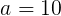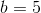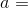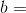# AP Computer Science A : Program Implementation

## Example Questions

← Previous 1 3 4 5 6 7 8

### Example Question #1 : Programming Constructs

Which of the following code samples adequately uses constants?

final int rows = 4;

for(int i = 0,l = rows; i < l; i++) {

rows += 2;

}

for(final int i = 0, rows = 5; i < rows; i++) {

System.out.println(i);

}

final int rows;

int intVal;

// Variable intVal read in during this code.... Excerpted...

rows = intVal;

for(int i = 0; i < rows; i++) {

System.out.println(i);

}

const int rows = 4;

for(int i = 0; i < rows; i++) {

System.out.println(i);

}

final String s = "Hello, "

int intVal;

// Variable intVal read in during this code.... Excerpted...

if(intVal < 0) {

s += "World!";

} else if(intVal == 0) {

s += "People!";

} else {

s += "Folks!";

}

final int rows;

int intVal;

// Variable intVal read in during this code.... Excerpted...

rows = intVal;

for(int i = 0; i < rows; i++) {

System.out.println(i);

}

Explanation:

Remember that a constant variable cannot be changed once it has been assigned.  Normally, you assign the variable immediately on the same line as the declaration.  However, if you were to create the constant and wait to assign a value, that would be find syntactically as well.  Thus, the correct answer does not have a problem, though it might appear so at first if you did not know this.  Now, remember that the way to declare a constant is to use the keyword "final".  (The keyword "const" works in some other languages like C++.)  All of the incorrect answers (other than the one using "const") alter the constant after it has been defined.

### Example Question #2 : Programming Constructs

In the following block of code, which of the following lines contains an error?

final int i = 20, j, k;

int l,m=50,n = 2;

j = n + m;

l = m * i + j;

for(int a = 0; a < m; a++) {

l += l;

}

m = 20 + j + n * i + m;

j = m + i;

k = 50 + 20 * j;

k = 50 + 20 * j;

for(int a = 0; a < m; a++)

l = m * i + j;

l += l;

j = m + i;

j = m + i;

Explanation:

The line

j = m + i;

has an error because it contains a reassignment to a constant (final) variable.  You are permitted to assign a constant on a line that is not the line of declaration.  However, once you do this, you cannot reassign a value.  The variable j was assigned a value on the line:

j = n + m;

Thus, the line above (j = m + i;) represents a re-assignment, hence causing an error.

### Example Question #1 : Variable Declarations

// ^ is Exclusive-Or (XOR) operator

x = x ^ y

y = x ^ y

x = x ^ y

What is the effect of the above snippet of code?

x is equal to y

The values are switched.

The values are the same.

The bits of x and y are negated.

None of the other answers is true.

The values are switched.

Explanation:

Consider two variables:(binary 1010) and(binary 0101). The exclusive or operator returns 1 in every bit that is different in the two numbers and returns 0 in every bit that is the same.

In order to switch the numbers, we must do this:^^^Continuing with the example,^would return 1111, because no bits are the same between the two numbers. Store this in the variable. Then 1111 ^ 0101 would return 1010. That result will be stored in. Finally, find the value of(1111) ^(1010) which is 0101, and store the result in variable. The values are now switched.

### Example Question #1 : Variable Declarations

Which of the following declares a String array of size i?  (Presume Java syntax.)

String[] s[i];

String s[] = new String[i];

None of the others

String s = new String[i];

String[] s = new String[i];

String[] s = new String[i];

Explanation:

For arrays, it is best to think about the array itself as being a type.  So, just as an int named x is declared:

int x;

And a String named s is declared:

String s;

You begin the declaration of a String array like this:

String[]

This is like saying, "What is coming next will be an array of String objects."

String[] s = new String[i];

This declares the new size as well, appropriately using the new operator.

### Example Question #3 : Variable Declarations

Which of the following code snippets declares an array of integers using an array literal?

int[] a = new int;

a = 42;

a = 26;

a = 134;

a = -13;

a = 45;

a = 234;

int[] a;

a = 42;

a = 26;

a = 134;

a = -13;

a = 45;

a = 234;

int[] a = {42,26,134,-13,45,234};

int a = {42,26,134,-13,45,234};

int[] a = {0:42,1:26,2:134,3:-13,4:45,5:234};

int[] a = {42,26,134,-13,45,234};

Explanation:

There are two things to pay attention to for this question.  First, you can make a simple array literal in Java by enclosing the list of array elements in a pair of curly braces.  Thus, the array literal

{42,26,134,-13,45,234}

is just fine!  However, you do not give the indices.  These are inferred.  (The first element is at 0, the second at 1, etc.)

Now, you just have to assign this to an array object.  Thus the following is wrong:

int a = {42,26,134,-13,45,234};

You need to have the [] to indicate that a is an array:

int[] a = {42,26,134,-13,45,234};

### Example Question #1 : Programming Constructs

What is the difference between double and int?

Int can hold all values whereas double can only store two-digit values.

Int can hold real numbers and double can only hold imaginary numbers.

Int can only hold integer values, double can hold any number including decimals.

There is no difference.

Int can hold positive numbers only and double can hold all numbers.

Int can only hold integer values, double can hold any number including decimals.

Explanation:

Int can only store integer values, whereas double can hold both integers and decimals, but at the same time uses more memory.

### Example Question #1 : Variable Declarations

int x=2;

double y=2.1;

float z=3.0;

int c=(x*y) + z;

What is the value of c

7

6

7.1

7.2

6.3

7

Explanation:

Remember that if a variable is declared as an integer, it can't have any decimals.

int c=(x*y) + z;

int c=(2*2.1)+3.0

From here, we do our math operations to solve, remembering to use the correct order of operations. Thus, start with the parentheses first then do the addition.

int c=4.2+3

int c= 4+3

int c=7

Any leftover fractions are cut off, so the answer is shortened to just 7.

### Example Question #6 : Variable Declarations

#include <iostream>

using namespace std;

int main()
{
string str("ComputerScience");
string mystr;
str = str + "SampleQuestionCS";
mystr = str.substr(7,8);

return 0;
}

What is the value of mystr after this code is run?

SampleQuestion

Science

rScience

ComputerS

QuestionCS

rScience

Explanation:

Two strings are created here: str and mystr.

In the 3rd line of main, str is concatenated with another string, making it even longer.

In the fourth line, the substr function is called. This function takes in 2 inputs, the starting index and the length of the string.

So, starting from index 7 of str, we get "r". Now, grabbing a total of 8 characters, including the "r", we get "rScience".

mystr="rScience".

### Example Question #7 : Variable Declarations

• Declare a string set to the the word cat.
• Declare a list of strings with the words hello, mama, and meow in it.
• Declare a hashmap of <string, string> <key, value> pairs with the word world having a value of earth.

String cat = "cat";

List<String> stringList = new ArrayList<String>();

stringList.add("hello");

stringList.add("mama");

stringList.add("meow");

HashMap<String, String> hashStrings = new HashMap<String, String>();

hashStrings.put("world", "earth");

List<String> stringList = new ArrayList<String>();

stringList.add("hello");

stringList.add("mama");

stringList.add("meow");

HashMap<String, String> hashStrings = new HashMap<String, String>();

hashStrings.put("world", "earth");

String cat = "cat";

List<String> stringList = new ArrayList<String>();

HashMap<String, String> hashStrings = new HashMap<String, String>();

hashStrings.put("world", "earth");

String cat;

List<String> stringList;

HashMap<String, String> hashStrings;

String cat = "cat";

List<String> stringList = new ArrayList<String>();

stringList.add("hello");

stringList.add("mama");

stringList.add("meow");

HashMap<String, String> hashStrings = new HashMap<String, String>();

hashStrings.put("world", "earth");

Explanation:

The correct answer defines all of the variables specified in the prompt. The answer with just the variable definitons is incorrect because it does not specify the declarations in the prompt. One the answers does not have the first variable defined so it is incorrect. And the final answer does not have the List variable declared, so it is also incorrect.

### Example Question #1 : Variable Declarations

Which of the following declares a String array of size i?  (Presume Java syntax.)

String[] s[i];

String s[] = new String[i];

None of the others

String s = new String[i];

String[] s = new String[i];

String[] s = new String[i];

Explanation:

For arrays, it is best to think about the array itself as being a type.  So, just as an int named x is declared:

int x;

And a String named s is declared:

String s;

You begin the declaration of a String array like this:

String[]

This is like saying, "What is coming next will be an array of String objects."